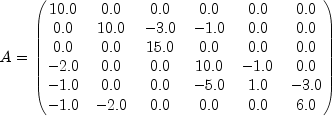JMSLTM Numerical Library 7.2.0
com.imsl.math

Class SparseMatrix.SparseArray

• Enclosing class:
SparseMatrix

public static class SparseMatrix.SparseArray
extends Object
The SparseArray class uses public fields to hold the data for a sparse matrix in the Java Sparse Array format. This format came about as a means for storing sparse matrices in Java. In this format, a sparse matrix is represented by two arrays of arrays. One array contains the references to the nonzero value arrays for each row of the sparse matrix. The other contains the references to the associated column index arrays.

As an example, consider the following real sparse matrix:In SparseArray, this matrix can be represented by the two jagged arrays, values and index, where values refers to the nonzero entries in A and index to the column indices:
double values[][] = { {10.0}, {10.0, -3.0, -1.0}, {15.0}, {-2.0, 10.0, -1.0}, {-1.0, -5.0, 1.0, -3.0}, {-1.0, -2.0, 6.0} }

int index[][] = { {0}, {1, 2, 3}, {2}, {0, 3, 4}, {0, 3, 4, 5}, {0, 1, 5} }

• Field Detail

• index

public int[][] index
Jagged array containing column indices. The length of this array equals numberOfRows. The length of each row equals the number of nonzeros in that row of the sparse matrix.
• numberOfColumns

public int numberOfColumns
Number of columns in the matrix.
• numberOfNonZeros

public long numberOfNonZeros
Number of nonzeros in the matrix.
• numberOfRows

public int numberOfRows
Number of rows in the matrix.
• values

public double[][] values
Jagged array containing sparse array values. This array must have the same shape as index.
• Constructor Detail

• SparseMatrix.SparseArray

public SparseMatrix.SparseArray()
JMSLTM Numerical Library 7.2.0